Courses

# Test: Shear Stresses in Beams

## 10 Questions MCQ Test Topicwise Question Bank for Mechanical Engineering | Test: Shear Stresses in Beams

Description
This mock test of Test: Shear Stresses in Beams for Mechanical Engineering helps you for every Mechanical Engineering entrance exam. This contains 10 Multiple Choice Questions for Mechanical Engineering Test: Shear Stresses in Beams (mcq) to study with solutions a complete question bank. The solved questions answers in this Test: Shear Stresses in Beams quiz give you a good mix of easy questions and tough questions. Mechanical Engineering students definitely take this Test: Shear Stresses in Beams exercise for a better result in the exam. You can find other Test: Shear Stresses in Beams extra questions, long questions & short questions for Mechanical Engineering on EduRev as well by searching above.
QUESTION: 1

Solution:
QUESTION: 2

Solution:
QUESTION: 3

### Select the WRONG statements:

Solution:

The moment of inertia of any area is always least with repsectto its center axis (not w.r.t radius of gyration).

QUESTION: 4

The shear stress distribution for a Particular section is shown as below: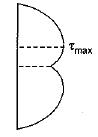The section is

Solution: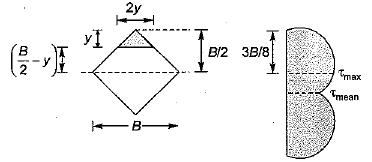Moment of inertia about neutral axis,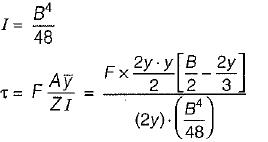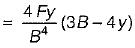QUESTION: 5

Shear center is the point in or outside a section through which the shear force applied produces only

Solution:

Shear center is the point in or outside a section through which the shear force applied produces no torsion or twist of the member.

QUESTION: 6

The section modulus of a circular section about an axis through its centre of gravity is

Solution: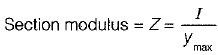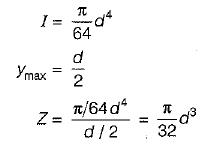QUESTION: 7

The distribution of shear stress of a beam is shown in the given figure.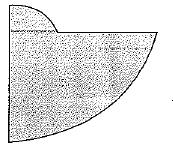The cross-section of the beam is

Solution: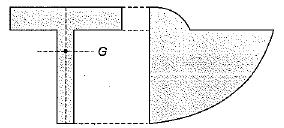QUESTION: 8

Select the correct shear stress distribution diagram for a square beam with a diagonal in a vertical position.

Solution:QUESTION: 9

The cross-section of a beam in bending is as shown in the figure below. It is subjected to a shear force acting in the plane of cross-section.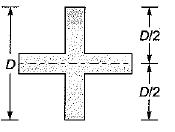Which among the following figure shows the correct shear stress distribution across the depth of the cross-section of the beam?

Solution: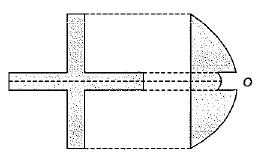The distribution will be parabolic and shear stress will suddenly drop at larger width point. In the larger width portion, it also varies parabolically.

QUESTION: 10

Which stress comes when there is an eccentric load applied?

Solution:

When there is an eccentric load it means that the load is at some distance from the axis. This causes compression in one side and tension on the other. This causes bending stress.